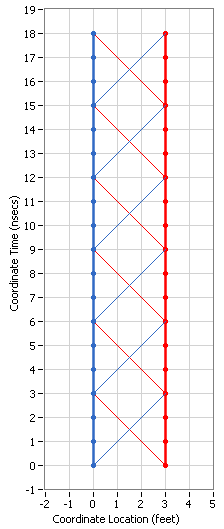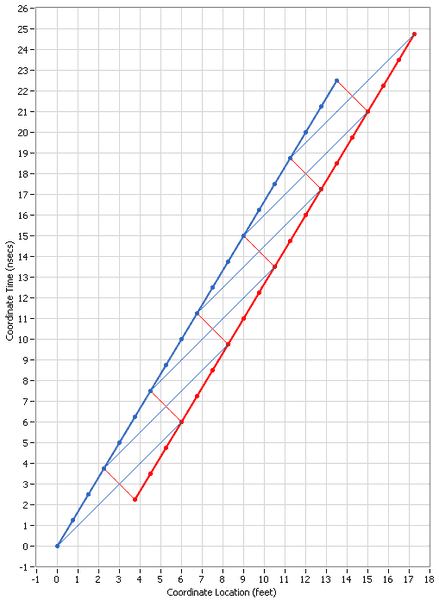# Time dilation and length contraction

suryanarayan
can both time dilation and length contraction occur for the same observer??? i dont think it is possible since then c=reduced length/increased time which is impossible(since c is constant)... Am i wrong?

Mentor
can both time dilation and length contraction occur for the same observer??? i dont think it is possible since then c=reduced length/increased time which is impossible(since c is constant)... Am i wrong?

Yes, they can and they do. You're mixing up the two different observers' times and distances.

Suppose I'm sitting on the earth, you're flying past me in a spaceship. A light signal is emitted at the rear of the spaceship, travels forward and reaches the nose. You look at the time of emission at the stern and the time of detection at the nose, subtract the one from the other to get the travel time, divide that into the length of the spaceship to get the speed of the light signal, and of course you get c.

Likewise, I look at the time of emission at the stern and the time of detection at the nose, subtract the one from the other to get the travel time. Because of time dilation, this will be a different and longer time than you measured. However, I do not divide that into the (contracted, for me) length of the spaceship to get the velocity. For me, the distance traveled is the distance between where the stern of the ship was when the light signal was emitted and where the nose was when the light signal was received - and because the ship was moving, that's longer than just the length of the spaceship. So I measure a longer travel time for the light signal, but I also see a longer distance traveled, despite the length contraction, so when I divide the time into the distance t get the speed, I also get c.

It's worth the effort of trying to draw a space-time diagram (many examples in other threads in this forum) to really understand how all of this hangs together.

Gold Member
can both time dilation and length contraction occur for the same observer??? i dont think it is possible since then c=reduced length/increased time which is impossible(since c is constant)... Am i wrong?
First off, I'd like to congratulate you for getting the definition of Time Dilation correct. A lot of people think that both time and length are reduced by the same amount and so they cancel out permitting the speed of light to remain at c for moving objects (they think). It's good that you see the problem.

The solution is that there is a third aspect of Special Relativity call Relativity of Simultaneity. Let me show you a spacetime diagram for a special light clock which has two impulses of light bouncing back and forth between two mirrors:Note how the two impulses strike opposite mirrors at exactly the same time.

Now we use the Lorentz Transformation process to see what this same light clock looks like in a frame in which it is moving at 0.6c:Note that the spacing between the mirrors is Length Contracted from 3 feet to 2.4 feet, as you stated.

Note that the ticks on the light clock are Time Dilated from 6 nsecs to 7.5 nsecs, as you correctly pointed out.

But also note that the light impulses don't strike the opposite mirrors at the same time. If they did, the propagation of light would have to be different in the two directions which is what you expressed concern about. Instead, light continues to propagate at c along the 45-degree angles and takes a different amount of time to travel in each direction but that is because the mirrors are moving and it has farther to go in one direction than in the other.

•